# Cloth: Representation for Simulations

There are many ways to model cloth! We'll explore continuous and discrete options to do so. We'll also dive deep into a discrete representation by reviewing how we can simulate cloth with particles and forces.

Keywords: cloth simulation, fabrics, numerical methods

Let’s continue our conversation about cloth after having already discussed the materials used to construct it. Although each piece of fabric is different, we can model the internal structures the same way.

According to scientific papers, cloth can be modeled either continuously or discretely. In this article, we focus on a discrete representation since we already have learned what mass-spring systems are ⚖️.

With this choice, we will dive into how to model fabric in a 3D environment - including the usage of triangles, particles and forces.

⚠️ My goal is to create a visually pleasing simulation. Exact physical accuracy to predict real-world scenarios is not included in this objective.

## Modeling - continuous vs discrete

We need to first choose how to model our fabric, as this will influence our entire simulation! Most research models fabric as either a continuous or discrete material.

### Continuous

A continuous representation ignores the fact that there are atoms and holes between the threads (between warps and wefts). In other words, the fabric is like a single body where a force or deformation acts entirely on this body. Consequently, if I choose a point anywhere on the fabric, it could exist because there are no discontinuities.

This approach opens the door to using continuum mechanics to simulate our cloth, which is described by Wikipedia as the following:

Continuum mechanics is a branch of mechanics that deals with the mechanical behavior of materials modeled as a continuous mass rather than as discrete particles.

This branch of mechanics allows for a more authentic and realistic simulation. However, the calculations are expensive and complex because of the systems of partial differential equations! 🧮

Additionally, during the simulation it would be necessary to discretize our model in time and space. To do this, one generally uses a finite element discretization using the finite element method (FEM).

The idea of FEM is to discretize, or to mesh, a continuous surface into simple elements, or meshes.⚠️ The discussion on this subject is too vast for this blog. Here are some resources that can help you.

### Discrete

A discrete representation is based on the fact that a piece of cloth is not continuous at all.

From our conversation from last week, we know that cloth is made up of intersecting threads (warps and wefts). Thus, if we zoom in on a piece of fabric, we will see its true structure 🧵 :🤔 Can you recognize the warp and weft weaves? If not, take a look at the article from last week to review!

As the fabric moves, threads collide, bend, etc due to the intertwining of the warps and wefts. It is therefore possible to model the threads as individual discrete elements. However, modeling all of these discrete elements is complex and impractical.

In this case, scientific researchers recommend a particle representation: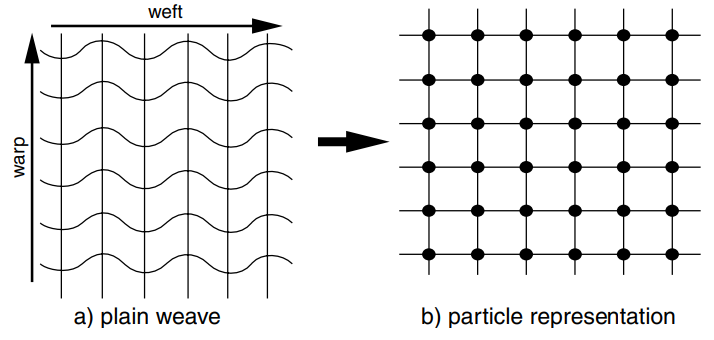This representation zooms in on the discrete element - the particle - which represents a crossing point between a weft and a warp. The mechanical interactions (the forces of stretching, compression, bending, and twisting) are calculated on the basis of the relations between neighboring local particles.

## The discrete representation of fabric

I am choosing to move forward with a discrete fabric representation since we’ve already implemented a particle system in the past. So, let’s dive a little deeper into this type of representation.

### Triangles

Our model will be a triangular mesh, where each vertex will be our representation of a particle. Here is an example: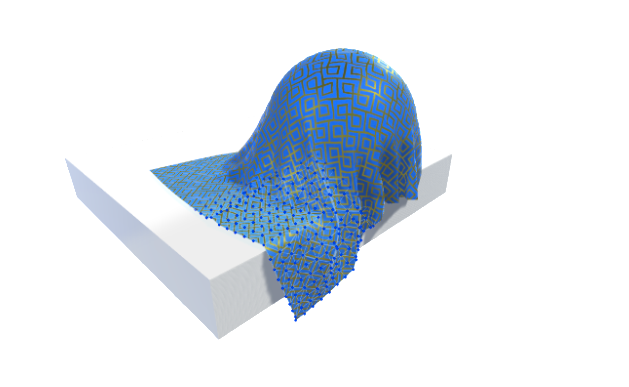by Obi

The blue points that are visible on the fabric are particles in this mesh! The fabric I just created in Blender is close to this idea: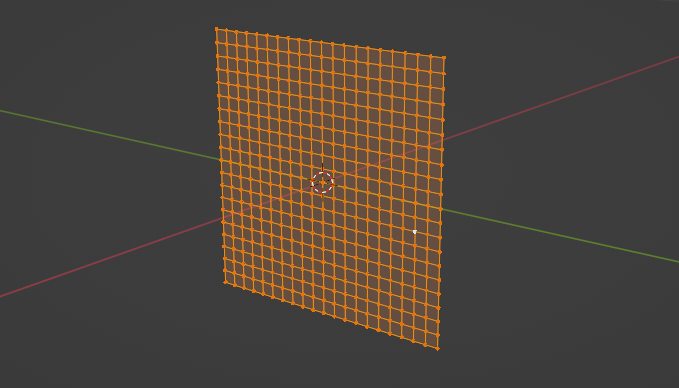When I export it, I’ll choose to triangulate the mesh to ensure I’m working with triangles.

### Particles

The behavior of particles is governed by the laws of physics which describe material properties. In other words, the particles must react to the forces acting on them.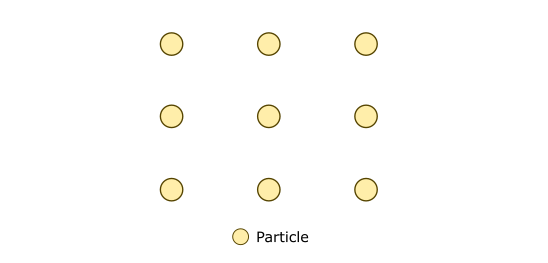To do this, the position and velocity of the particles must change over time. The combination of position and velocity in a data structure is called phase space. We have already seen this representation of particles a few months ago during the article on the creation of a system of particles.

### The mass

In the next part, we will see that particles are part of a mass-spring system. That said, how is the mass of a given particle determined? 🤔

Since the fabric is formed by a mesh of triangles, one option for calculating the mass is to first define a density for the fabric. Recall that the unit of density is kg/m². Therefore, we need to find the area of a triangle to find its mass. Finally, we divide this total mass by three to find the mass of a vertex.

Let’s see a fun trick to find the area of a triangle using the cross product. The magnitude of the product u × v is by definition the area of the parallelogram. Therefore, we can use the cross product to calculate the area of a triangle formed by three points A, B, and C in space.

Suppose we know the position of each vertex. It is thus possible to solve the area as follows: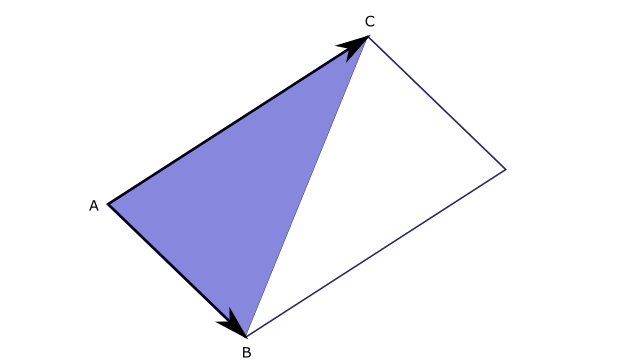$A = \frac{1}{2}|\vec{AB} \times \vec{AC}|$

### The forces

Although there are external forces that affect fabric such as gravity, wind and collisions with other bodies, internal forces are responsible for the behavior of cloth as we know it.

The internal forces of interest that will act on the particles in our model are stretch, bend and shear. The idea is that these forces will cause the particles to move in a manner similar to how a fabric deforms and drapes.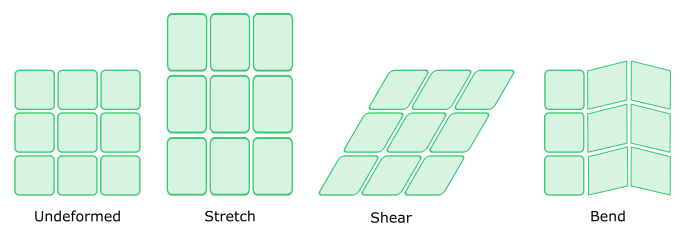The process of simulating such forces is the same as the 2D simulation we did together a few months ago.

We can model the interactions between particles as a mass-spring system. Here is an example of such a system: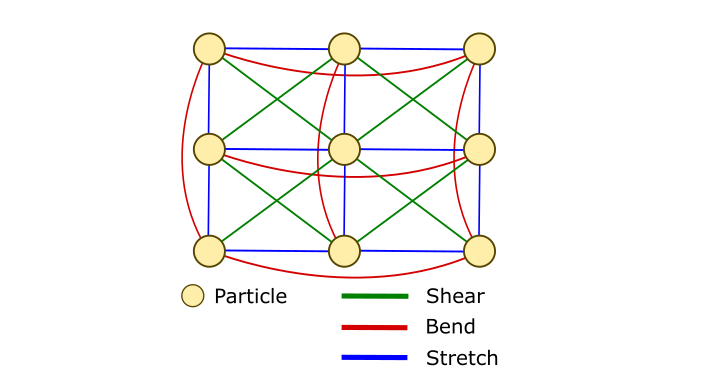We just need to calculate the position and the velocity of each particle after taking into account all the forces. We must use a numerical method to solve this system as we have already seen in a previous article - Implicit Euler method.

In any case, you must choose the numerical method that meets your needs.

## Next time

We’re going to build off this knowledge and take a deeper dive into a potential way to solve this cloth simulation, with position based dynamics (PBD).

## Comments for Cloth: Representation for Simulations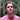Written by Carmen Cincotti, computer graphics enthusiast, language learner, and improv actor currently living in San Francisco, CA.  Follow @CarmenCincotti